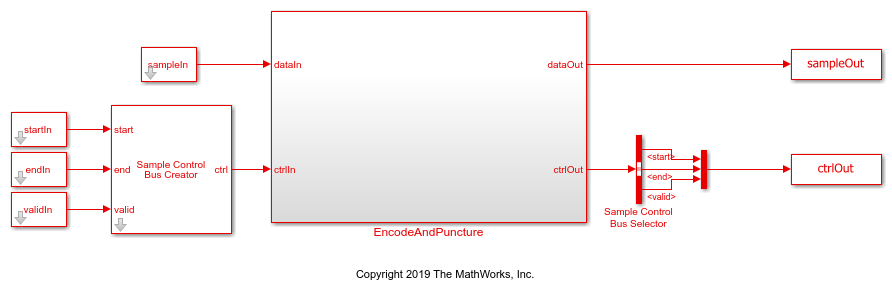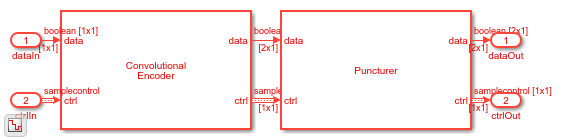# Convolutional Encode and Puncture Streaming Samples

This example shows how to use the hardware-friendly Convolutional Encoder and Puncturer blocks to encode samples at WLAN code rates.

1. Generate random input frame samples with frame control signals by using the `whdlFramesToSamples` function in MATLAB®.

2. Import these samples into a Simulink® model and run the model to encode and puncture the samples.

3. Export the result of the Simulink simulation back to MATLAB.

4. Generate reference samples using the `convenc` MATLAB function with puncturing enabled.

5. Compare the Simulink results with the reference samples.

The example model supports HDL code generation for the `EncodeAndPuncture` subsystem, that contains the Convolutional Encoder and Puncturer blocks.

```modelname = 'GenConvEncPuncturerModel'; open_system(modelname); ```Set up workspace variables that describe the code rate. The Convolutional Encoder block supports constraint lengths in the range [3,9] and polynomial lengths in the range [2,7].

Starting from a code rate of 1/2, IEEE 802.11 WLAN specifies three puncturing patterns to generate three additional code rates. Choose one of these code rates, and then set the frame size and puncturing pattern based on that code rate. You can also choose the unpunctured code rate of 1/2.

IEEE 802.11 WLAN specifies different code rates and uses `'Terminated'` mode. The blocks also support `'Continuous'` mode and `'Truncated'` modes, but they are not included in this example.

```constraintLength = 7; codeGenerator = [133 171]; trellis = poly2trellis(constraintLength,... codeGenerator); % IEEE 802.11n-2009 WLAN 1/2 (7, [133 171]) % Rate Puncture Pattern Maximum Frame Size % 1/2 [1;1;1;1] 2592 % 2/3 [1;1;1;0] 1728 % 3/4 [1;1;1;0;0;1] 1944 % 5/6 [1;1;1;0;0;1;1;0;0;1] 2160 codeRate = 3/4; if (codeRate == 2/3) puncVector = logical([1;1;1;0]); frameSize = 1728; elseif (codeRate == 3/4) puncVector = logical([1;1;1;0;0;1]); frameSize = 1944; elseif (codeRate == 5/6) puncVector = logical([1;1;1;0;0;1;1;0;0;1]); frameSize = 2160; else % codeRate == 1/2 puncVector = logical([1;1;1;1]); frameSize = 2592; end ```

Generate input frame samples for encoding and puncturing by using Communications Toolbox™ System objects to generate encoded samples.

```numFrames = 5; txMessages = cell(1,numFrames); txCodeword = cell(1,numFrames); for ii = 1:numFrames txMessages{ii} = logical(randn(frameSize-constraintLength+1,1)); end ```

Set up variables for Simulink simulation. The Simulink model requires streaming samples with accompanying control signals. Calculate the required simulation time from the latency of the Convolutional Encoder and Puncturer blocks.

```samplesizeIn = 1; idlecyclesbetweensamples = 0; idlecyclesbetweenframes = constraintLength-1; [sampleIn,ctrlIn] = whdlFramesToSamples(txMessages, ... idlecyclesbetweensamples,idlecyclesbetweenframes,samplesizeIn); startIn = ctrlIn(:,1); endIn = ctrlIn(:,2); validIn = ctrlIn(:,3); simTime = size(ctrlIn,1)+6; sampletime = 1; ```

```set_param([modelname '/EncodeAndPuncture'],'Open','on'); sim(modelname); ```Convert the streaming samples from the Simulink block output to framed data for comparison.

```sampleOut = squeeze(sampleOut); startOut = ctrlOut(:,1); endOut = ctrlOut(:,2); validOut = ctrlOut(:,3); idxStart = find(startOut.*validOut); idxEnd = find(endOut.*validOut); ```

Generate reference samples using `convenc` MATLAB function.

```for ii = 1:numFrames txCodeword{ii} = convenc([txMessages{ii};false(constraintLength-1,1)],... trellis,puncVector); end ```

Compare the output samples against the generated input samples.

```fprintf('\nEncoded Samples\n'); for ii = 1:numFrames idx = idxStart(ii):idxEnd(ii); idxValid = (validOut(idx)); dataOut = sampleOut(:,idx); hdlTxCoded = dataOut(:,idxValid); numBitsErr = sum(xor(txCodeword{ii},hdlTxCoded(:))); fprintf('Number of samples mismatched in the frame #%d: %d bits\n',ii,numBitsErr); end ```
```Encoded Samples Number of samples mismatched in the frame #1: 0 bits Number of samples mismatched in the frame #2: 0 bits Number of samples mismatched in the frame #3: 0 bits Number of samples mismatched in the frame #4: 0 bits Number of samples mismatched in the frame #5: 0 bits ```